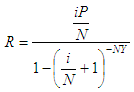# Calculate Loan Payment (Regular Payments on a Loan)

 We’re implementing here an algorithm in Matlab to calculate loan payment. This program calculates the amount required as regular payments in order to repay a loan over a specified time period. The specifications or conditions you must provide are the amount of the principal, the interest rate charged, the number of payments to be made per year and the number of years to pay.

This code assumes all installment payments will be equal.

The calculation is based on this formula:where:
R = regular payment
i = annual interest rate
P = principal
N = number of payments per year
Y = number of years

We show now a video about how to use the formula. After the video, we show you how to create a function to replicate the concept.

We then create a Matlab function to calculate the formula above:

function rpl = loan_payment(it, p, n, y)
it = it/100;
rpl = it*p/n /(1 - (it/n + 1)^(-n * y));

We create another script to test and drive the above m-file:

clc; clear; close all; format bank; format compact

y = input('Enter term in years: ');
p = input(
'Enter principal: ');
it = input(
'Enter annual interest rate: ');
n = input(
'Enter number of payments per year: ');

rpl = loan_payment(it, p, n, y)

Example 1:

What must you pay on a loan of \$4000 at 8% if payments are to be made quarterly for five years?

We run our driving code and enter:

Enter term in years: 5
Enter principal: 4000
Enter annual interest rate: 8
Enter number of payments per year: 4

The result is:

rpl = 244.63

Example 2:

If Donald borrows \$6500 at 12.5% from Illusions Rate Savings & Loan to be paid back over a period of 5.5 years, what would his monthly payments be?

Again, we run our code and enter:

Enter term in years: 5.5
Enter principal: 6500
Enter annual interest rate: 12.5
Enter number of payments per year: 12

The result is:

rpl = 136.68